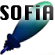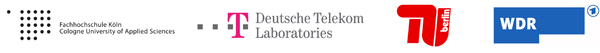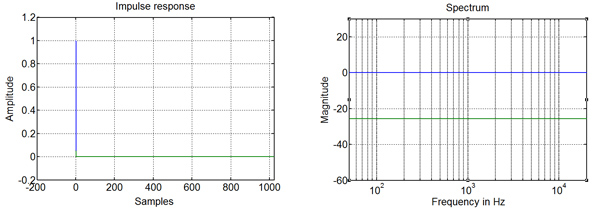Welcome to SOFiA
Who is behind SOFiA
Feature overview
System overview
Function reference
mergeArrayData
F/D/T
gauss
lebedev
S/W/G
S/T/C
W/G/C
S/F/E
M/F
R/F/I
P/D/C
I/T/C
makeMTX
makeIR
visual3D
Coordinate System
Application Examples
Example 1
Example 2
Example 3
Example 4
Example 5
Example 6
Example 7
Example 8
Array Datasets
VariSphear system
Groups and Mailinglists
Contact and Support
How to Reference

# SOFiA application example 6

In this example we generate an ideal full audio spectrum unity plane wave of order N=5 impinging to a rigid sphere array using I/W/G. We render impulse responses for two different pwd directions - the first one is exactly aligned towards the wave, the other one aims to one side. A rejection of >20dB can be observed for the second one.

## File(s)

Run `sofiaAE6.m`.

## Output## Code

 ``` /* % SOFiA example 6: Impulse response reconstruction on a simulated ideal unity plane wave. % SOFiA Version : R11-1220 clear all clc % Generate a full audio spectrum plane wave using I/W/G ``` ` ` ` ` ``` Nwave = 5;Wave order r = 1; % Array radius ac = 2; % Array configuration: 2-Rigid Sphere FS = 48000; % Sampling Frequency NFFT = 1024; % FFT-Bins AZ = 0; % Azimuth angle EL = pi/2; % Elevation Angle [Pnm, kr] = sofia_wgc(Nwave, r, ac, FS, NFFT, AZ, EL); `````` ``` ``` % Make radial filters Nrf = Nwave; % radial filter order dn = sofia_mf(Nrf, kr, ac); % Running a plane wave decomposition for different look directions Npdc = Nwave; % Decomposition orde OmegaL = [0 pi/2; pi/2 pi/2]; Looking towards the wave and to one side Y = sofia_pdc(Npdc, OmegaL, Pnm, dn); impulseResponses = sofia_makeIR(Y); % Reconstruct impulse responses figure(1) subplot(1, 2, 1) plot('impulseResponses'); title('Impulse response'); xlabel('Samples'); ylabel('Amplitude'); axis([-200 NFFT -0.2 1.2]) grid on % Plot results (Spectra) spectrum = abs(fft('impulseResponses')); fscale = FS/2*linspace(0,1,NFFT/2+1); subplot(1; 2; 2) semilogx(fscale,20*log10(spectrum(1:end/2+1,:))) title('Spectrum'); xlabel('Frequency in Hz'); ylabel('Magnitude'); axis([50 20000 -60 30) grid on ``` `*/`